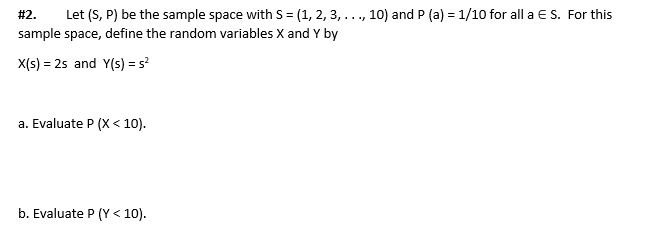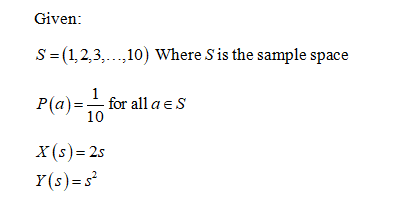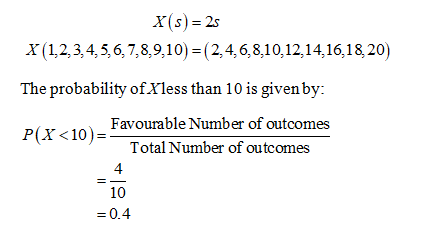# Let (S, P) be the sample space with S = (1, 2, 3, ..., 10) and P (a) = 1/10 for all a ES. For thissample space, define the random variables X and Y by#2.X(s) = 25 and Y(s) = sa. Evaluate P (X< 10).b. Evaluate P (Y< 10).

Question
1 viewshelp_outlineImage TranscriptioncloseLet (S, P) be the sample space with S = (1, 2, 3, ..., 10) and P (a) = 1/10 for all a ES. For this sample space, define the random variables X and Y by #2. X(s) = 25 and Y(s) = s a. Evaluate P (X< 10). b. Evaluate P (Y < 10). fullscreen
check_circle

Step 1Step 2...

### Want to see the full answer?

See Solution

#### Want to see this answer and more?

Solutions are written by subject experts who are available 24/7. Questions are typically answered within 1 hour.*

See Solution
*Response times may vary by subject and question.
Tagged in

### Probability